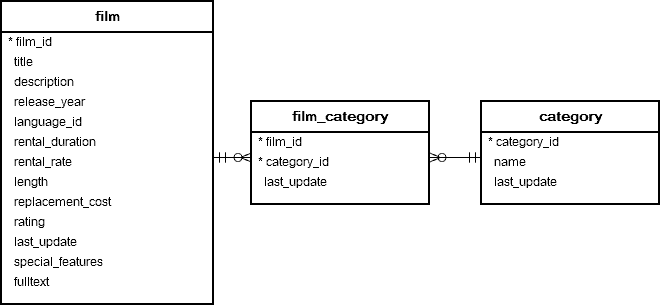# PostgreSQL Tutorial: TRUNC Function: Truncate Numbers to a Specified Decimal Places

August 7, 2023

The PostgreSQL `TRUNC()` function returns a number truncated to a whole number or truncated to the specified decimal places.

## Syntax

The following illustrates the syntax of the PostgreSQL `TRUNC()` function:

``````TRUNC(number [, precision])
``````

## Arguments

The `TRUNC()` function accepts two arguments.

1) `number`

The `number` argument is a numeric value to be truncated

2) `precision`

The `precision` argument is an integer that indicates the number of decimal places.

If the `precision` argument is a positive integer, the `TRUNC()` function truncates digits to the right of the decimal point.

In case the `precision` is a negative integer, the `TRUNC()` function replaces digits to the left of the decimal point.

The precision argument is optional. If you don’t specify it, it defaults to zero (0). In other words, the `number` is truncated to a whole number.

## Return value

The PostgreSQL `TRUNC()` function returns the same numeric data type as the first argument if the second argument is not specified. Otherwise, the function returns a numeric value if both arguments are used.

## Examples

### A) Truncate to a whole number example

The following example uses the `TRUNC()` function to truncate a number to an integer:

``````SELECT
TRUNC(10.6);
``````

The result is:

``````10
``````

### B) Truncate to the specified decimal place

The following statement truncates a number to 2 decimal places:

`````` SELECT
TRUNC(
1.234,
2
);
``````

Here is the result:

``````1.23
``````

### C) Truncate numbers with negative second argument example

Consider the following example:

``````SELECT
TRUNC(150.45,-2)
``````

The second argument is -2, therefore, the `TRUNC()` function replaced the digits to the left of the decimal point that results in:

``````100
``````

### D) Truncate numbers returned by a query

See the following `film`, `film_category`, and `category` tables in the sample database:The following statement calculates the average rental rate by film category:

``````SELECT
NAME,
TRUNC(AVG( rental_rate ),2)
FROM
film
INNER JOIN film_category
USING(film_id)
INNER JOIN category
USING(category_id)
GROUP BY
NAME
ORDER BY NAME;
``````

In this example, we used the `TRUNC()` function to truncate the average rentals to two decimal places.

The following picture illustrates the result:In this tutorial, you have learned how to use the PostgreSQL `TRUNC()` function to truncate numbers.